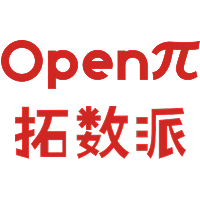# 云上 Index：看「简墨」如何为云原生打造全新索引2023-08-01## 1 存储的详细设计

• 持久层：持久层是 PieCloudDB 中的底层存储，通常采用分布式文件系统或对象存储系统 等云原生存储，如 AWS S3、Azure Blob Storage 等。持久层具有高可用性、持久性等特点，能够安全地保持数据并保证数据的长期存储。
• 数据层：数据层是 PieCloudDB 的上层抽象，提供面向应用程序的标准访问接口，包括半结构化数据、结构化数据和支持 SQL 的无结构化数据存储。

1.1 PieCloudDB 数据的持久化设计

• N 元存储模型（即通常所说的行存）
• 分解存储模型（即通常所说的列存）
• 混合存储模型

PieCloudDB 采用了第三种：混合存储模型。混合存储模型是将一组数据水平分组，然后将它们的属性垂直划分为列。通过这样的存储模型，PieCloudDB 得以获得列式存储高效处理和压缩友好等优势，同时保留行存储的空间局部性优势，降低数据重组的开销。这一存储模型的选择也影响了 PieCloudDB 中的索引设计，后文将详细介绍。

1.2 PieCloudDB 存储底座

PieCloudDB 使用对象存储技术作为云原生虚拟数仓存储底层。对象存储可以带来可伸缩性、弹性扩展和高度容错性等优点。然而，在实际的使用过程中往往也遇到一些限制，主要包括以下几个方面：

• 延迟：与传统的块存储技术相比，对象存储技术往往具有较高的延迟，因此在某些应用场景下，可能会对数据库的性能产生一定影响。
• 大规模重写操作难以支持：对象存储基于分布式系统实现，而复杂的存储操作（如大规模数据的重写）实现起来是比较困难的，但是，这类操作在关系型数据库中常常会遇到。
• 事务管理：对象存储通常提供了乐观并发控制等简单的事务管理机制，但是它们在处理分布式事务时很复杂，并且由于分散在多处，难以跨所有节点维护一个全局锁或者其他的协调机制。
• 数据一致性：尽管对象存储具有高可靠性和冗余性，但其异步特性意味着分布式数据的一致性需要通过额外的手段来维护，远比其他的分布式数据库解决方案更为复杂，同时也存在较高的管理成本。

PieCloudDB 在存储的打造过程中进行了大量设计来弥补这些限制，保证用户的使用体验。例如针对其中第二个方面，PieCloudDB 的持久化文件在生成后无法进行原地修改。因此，PieCloudDB 在 update/delete 删除时，会生成新的文件，在新文件中将包含未修改的数据和新增的修改后的数据，并将保留旧的数据文件。相关细节将在未来的技术文章中进行说明，欢迎关注。

## 2 PieCloudDB 中的索引

• 使用混合存储模型
• 持久化的文件不会被修改

2.1 索引的常见类型

2.2 PieCloudDB 中的 Data Skipping 索引

Zone Map 索引和 BRIN（Block Range INdex）索引是在 OLAP 场景中常见的数据跳跃（Data Skipping）技术的具体实现方式。它们都利用了预先计算的统计信息来跳过不符合查询条件的数据块，从而加速查询的执行。

Zone Map 是通过存储每个数据块的选定列的预先计算统计信息，例如最小值和最大值，以及其他聚合信息。在查询期间，数据库可以使用这些统计信息来裁减要访问的数据块，从而减少不必要的 I/O 操作，提高查询性能。这在 OLAP 场景中对大规模数据集的查询非常有用。

2.3 示例

```create table dataskip (a int, b int);
insert into dataskip select i, i*2 from generate_series(1, 1000)i;
insert into dataskip select i, i*2 from generate_series(1001, 2000)i;
insert into dataskip select i, i*2 from generate_series(2001, 3000)i;
insert into dataskip select i, i*2 from generate_series(3001, 4000)i;
insert into dataskip select i, i*2 from generate_series(4001, 5000)i;
insert into dataskip select i, i*2 from generate_series(5001, 6000)i;
insert into dataskip select i, i*2 from generate_series(6001, 7000)i;
insert into dataskip select i, i*2 from generate_series(7001, 8000)i;
insert into dataskip select i, i*2 from generate_series(8001, 9000)i;
insert into dataskip select i, i*2 from generate_series(9001, 10000)i;
```

```demo=# explain analyze select * from dataskip where a < 10;
QUERY PLAN
---------------------------------------------------------------------------------
Gather Motion 3:1 (slice1; segments: 3) (cost=2.00..10.21 rows=3 width=8) (actual time=34.361..36.928 rows=9 loops=1)
-> Bitmap Heap Scan on dataskip (cost=2.00..10.17 rows=1 width=8) (actual time=16.189..31.790 rows=5 loops=1)
Recheck Cond: (a < 10)
Rows Removed by Index Recheck: 316
-> Bitmap Index Scan on dataskip (cost=0.00..2.00 rows=333 width=0) (actual time=2.908..2.910 rows=1 loops=1)
Index Cond: (a < 10)
Planning Time: 4.259 ms
(slice0) Executor memory: 159K bytes.
(slice1) Executor memory: 32972K bytes avg x 3 workers, 32972K bytes max (seg0).
Memory used: 128000kB
Optimizer: Postgres query optimizer
Execution Time: 55.895 ms
(12 rows)
```

```demo=# set enable_bitmapscan = off;
SET
demo=# explain analyze select * from dataskip where a < 10;
QUERY PLAN
---------------------------------------------------------------------------------
Gather Motion 3:1 (slice1; segments: 3) (cost=0.00..51.71 rows=3 width=8) (actual time=129.916..140.925 rows=9 loops=1)
-> Seq Scan on dataskip (cost=0.00..51.67 rows=1 width=8) (actual time=2.939..132.546 rows=5 loops=1)
Filter: (a < 10)
Rows Removed by Filter: 3292
Planning Time: 0.099 ms
(slice0) Executor memory: 123K bytes.
(slice1) Executor memory: 32825K bytes avg x 3 workers, 32825K bytes max (seg0).
Memory used: 128000kB
Optimizer: Postgres query optimizer
Execution Time: 154.416 ms
(10 rows)
```

```demo=# explain analyze select * from dataskip join jtbl on dataskip.a = jtbl.a and jtbl.a < 10;
QUERY PLAN
---------------------------------------------------------------------------------
Gather Motion 3:1 (slice1; segments: 3) (cost=2.00..15.47 rows=3 width=16) (actual time=33.638..33.712 rows=9 loops=1)
-> Nested Loop (cost=2.00..15.43 rows=1 width=16) (actual time=33.300..33.405 rows=5 loops=1)
Rows Removed by Join Filter: 20
-> Redistribute Motion 3:3 (slice2; segments: 3) (cost=0.00..5.21 rows=2 width=8) (actual time=0.003..0.013 rows=5 loops=1)
Hash Key: jtbl.a
-> Seq Scan on jtbl (cost=0.00..5.17 rows=2 width=8) (actual time=3.144..20.979 rows=3 loops=1)
Filter: (a < 10)
Rows Removed by Filter: 356
-> Materialize (cost=2.00..10.19 rows=1 width=8) (actual time=5.547..5.554 rows=4 loops=6)
-> Redistribute Motion 3:3 (slice3; segments: 3) (cost=2.00..10.19 rows=1 width=8) (actual time=33.130..33.269 rows=5 loops=1)
-> Bitmap Heap Scan on dataskip (cost=2.00..10.17 rows=1 width=8) (actual time=11.766..24.910 rows=5 loops=1)
Recheck Cond: (a < 10)
Rows Removed by Index Recheck: 316
-> Bitmap Index Scan on dataskip (cost=0.00..2.00 rows=333 width=0) (actual time=2.783..2.784 rows=1 loops=1)
Index Cond: (a < 10)
Planning Time: 6.522 ms
(slice0) Executor memory: 220K bytes.
(slice1) Executor memory: 79K bytes avg x 3 workers, 80K bytes max (seg0). Work_mem: 17K bytes max.
(slice2) Executor memory: 32826K bytes avg x 3 workers, 32826K bytes max (seg0).
(slice3) Executor memory: 32975K bytes avg x 3 workers, 32975K bytes max (seg0).
Memory used: 128000kB
Optimizer: Postgres query optimizer
Execution Time: 68.989 ms
(25 rows)
```

```demo=# set enable_bitmapscan = off;
SET
demo=# explain analyze select * from dataskip join jtbl on dataskip.a = jtbl.a and jtbl.a < 10;
QUERY PLAN
---------------------------------------------------------------------------------Gather Motion 3:1 (slice1; segments: 3) (cost=0.00..56.97 rows=3 width=16) (actual time=139.811..139.886 rows=9 loops=1)
-> Nested Loop (cost=0.00..56.92 rows=1 width=16) (actual time=139.587..139.691 rows=5 loops=1)
Rows Removed by Join Filter: 20
-> Redistribute Motion 3:3 (slice2; segments: 3) (cost=0.00..5.21 rows=2 width=8) (actual time=0.003..0.011 rows=5 loops=1)
Hash Key: jtbl.a
-> Seq Scan on jtbl (cost=0.00..5.17 rows=2 width=8) (actual time=1.758..21.023 rows=3 loops=1)
Filter: (a < 10)
Rows Removed by Filter: 356
-> Materialize (cost=0.00..51.69 rows=1 width=8) (actual time=23.262..23.269 rows=4 loops=6)
-> Redistribute Motion 3:3 (slice3; segments: 3) (cost=0.00..51.69 rows=1 width=8) (actual time=136.260..139.557 rows=5 loops=1)
-> Seq Scan on dataskip (cost=0.00..51.67 rows=1 width=8) (actual time=1.730..134.913 rows=5 loops=1)
Filter: (a < 10)
Rows Removed by Filter: 3292
Planning Time: 0.248 ms
(slice0) Executor memory: 185K bytes.
(slice1) Executor memory: 79K bytes avg x 3 workers, 80K bytes max (seg0). Work_mem: 17K bytes max.
(slice2) Executor memory: 32826K bytes avg x 3 workers, 32826K bytes max (seg0).
(slice3) Executor memory: 32827K bytes avg x 3 workers, 32827K bytes max (seg0).
Memory used: 128000kB
Optimizer: Postgres query optimizer
Execution Time: 155.026 ms
(23 rows)
```

2.4 Primary Key 的支持

• 加速点查
• 确保唯一性约束
• 确保非空约束

## 3 PieCloudDB 的索引探索之路

3.1 基于 Data Skipping 思路的其他索引探索。

• 基于 bitmap 的索引
• bloom filter
• column sketchs
• column imprints
• ......

3.2 OLTP 场景下的索引